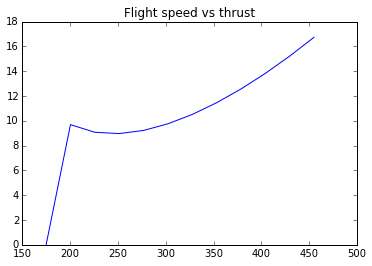# Chapter9-External Incompressible Viscous Flow¶

## Ex1-pg414¶

In :
import math
##Lengh of side of the test section(in mm):
L=305.;
##Freesteam speed at section 1(in m/sec):
U1=26.;
##Displacement thickness at section 1(in mm):
d1=1.5;
##Displacment thickness at section 2(in mm):
d2=2.1;
##static pressure##
#Change in static pressure between sections 1 and 2:
C=(((L-2.*d1)/(L-2.*d2))**4.-1)*100.;
print("RESULTS")
print'%s %.2f %s'%("Change in static pressure between the sections 1 and 2: ",C," percent ")

RESULTS
Change in static pressure between the sections 1 and 2:  1.61  percent


## Ex4-pg428¶

In :
import math
import scipy
from scipy import integrate
##Veocity of flow(in m/sec):
U=1.;
##Length of flat plate(in m):
L=1.;
##Density of water(in kg/m**3):
d=999.;
##Kinematic viscosity of water(in m**2/sec):
v=10**-6.;
##Displacement thickness and stress##
#Reynolds number:
ReL=U*L/v
##FOR TURBULENT FLOW
##Disturbance thickness(in m):
dL1=0.382/ReL**0.2*L
##Displacement thickness(in m):
def fun(n):
y=dL1*(1.-n**(1./7.))
return y

##Skin friction coefficient:
Cf1=0.0594/ReL**0.2
##Wall shear stress(in N/m**2):
tw1=Cf1*0.5*d*U**2
##For LAMINAR FLOW:
##Disturbance thickness(in m)
dL2=5./math.sqrt(ReL)*L
##Displacement thickness(in m):
dl2=0.344*dL2
##Skin friction coefficient:
Cf2=0.664/math.sqrt(ReL)
##Wall shear stress(in N/m**2):
tw2=Cf2*0.5*d*U**2
##COMPARISON OF VALUES WITH LAMINAR FLOW
##Disturbance thickness
D=dL1/dL2
##Displacement thickness
DS=dl1/dl2
##Wall shear stress
WSS=tw1/tw2
print("RESULTS")
print'%s %.2f %s'%("Disturbace thickness: ",dL1," m")
print'%s %.2f %s'%("Displacement thickness: ",dl1," m")
print'%s %.2f %s'%("Wall shear stress: ",tw1," N/m^2")
print("COMPARISON WIH LAMINAR FLOW\n")
print'%s %.2f %s'%(" Disturbance thicknes: ",D," ")
print'%s %.2f %s'%("Displacement thickness: ",DS,"")
print'%s %.2f %s'%("Wall shear stress: ",WSS," ")

RESULTS
Disturbace thickness:  0.02  m
Displacement thickness:  0.00  m
Wall shear stress:  1.87  N/m^2
COMPARISON WIH LAMINAR FLOW

Disturbance thicknes:  4.82
Displacement thickness:  1.75
Wall shear stress:  5.64


## Ex5-pg436¶

In :
import math
##Length of the supertanker(in m):
L=360.;
##Width of supertanker(in m):
W=70.;
##Draft of the supertanker(in m):
D=50.;
##Cruising speed in water(in knots):
s=13.;
##Kinematic viscosity at 10 C
v=1.37*10**-6;
##Density of sea water(in kg/m^3):
d=1020.;
##force and power##
##Speed in m/s:
U=s*6076.*0.305/3600.
##Reynolds number:
Re=U*L/v
##Drag coefficient:
Cd=0.455/math.log10(Re)**2.58-1610./Re
##Area(in m^2):
A=L*(W+D)
##Drag force(in N)
Fd=Cd*A*0.5*d*U**2
##Power required to overcome skin friction drag(in W):
P=Fd*U
print("RESULTS")
print'%s %.2f %s'%("Drag force:",Fd," N")
print'%s %.2f %s'%("Power required to overcome skin friction drag: ",P," W")

RESULTS
Drag force: 1444953.76  N
Power required to overcome skin friction drag:  9669686.75  W


## Ex6-pg442¶

In :
##Diameter of chimney(in m):
D=1.;
##Height of chimney(in m):
L=25.;
##Speed of wind(in kmph):
s=50.;
##Density of air(in kg/m^3):
d=1.23;
##Viscosity of air(in kg/(m-s)):
u=1.79*10**-5;
##Pressure(in kPa):
p=101.;

##Bending moment##
##filename=pathname+filesep()+'9.06-data.sci'
#exec(filename)
##Velocity in m/sec:
V=s*5./18.
##Reynolds number:
Re=d*V*D/u
##Value of Cd is obtained as:
Cd=0.35;
##Area(in m^2):
A=L**2;
##Moment about the chimney base(in N-m):
M0=Cd*A*D/4.*d*V**2
print("RESULTS")
print'%s %.2f %s'%("Bending moment at the bottom of the chimney: ",M0," N-m")

RESULTS
Bending moment at the bottom of the chimney:  12975.62  N-m


## Ex7-pg443¶

In :
##Weight of the dragster(in lbf):
w=1600.;
##Speed of dragster(in mph):
s1=270.;
##Area of drag chute(in ft^2):
A=25.;
##Speed of dragster after deceleraton(in mph):
s2=100.;
##Acceleration due to gravity(in ft/sec^2):
g=32.2;
##Density of air(in slug/ft^3):
d=0.00238;
##Value of coefficient of drag:
Cd=1.42;
##Time required##
#pathname=get_absolute_file_path('9.07.sce')
#filename=pathname+filesep()+'9.07-data.sci'
#exec(filename)
##Time required to decelerate to 100 mph(in seconds):
t=(s1-s2)*2.*w/(s1*s2)/Cd/d/A/g*3600./5280.
print("RESULTS")
print'%s %.2f %s'%("Time required to decelerate to 100 mph: ",t," seconds")

RESULTS
Time required to decelerate to 100 mph:  5.05  seconds


## Ex8-pg458¶

In :
import numpy
%matplotlib inline

import warnings
warnings.filterwarnings('ignore')
import math
import matplotlib
from matplotlib import pyplot
##Wing area(in ft^2):
A=1600.;
##Aspect ratio:
ar=6.5;
##Groos weight of aircraft(in lbf):
W=150000.;
##Coefficient of drag at zero lift :
Cd0=0.0182;
##Sonic speed at sea level(in mph):
c=759.;
##Density of air(in slug/ft^3):
p=0.00238;
##Srall speed at sea level(in mph):
Vssl=175.;

##Optimum cruise speed##
#pathname=get_absolute_file_path('9.08.sce')
#filename=pathname+filesep()+'9.08-data.sci'
#exec(filename)
##Plotting velocity with drag force
V=numpy.linspace(175,455,num=12);

n=len(V);
CL=numpy.zeros(n)
Cd=numpy.zeros(n)
Fd=numpy.zeros(n)
FD=numpy.zeros(n)
for i in range(1,n):
CL[i]=2*W/p*(3600/V[i]/5280.)**2/A;
Cd[i]=Cd0+CL[i]**2/math.pi/ar;
Fd[i]=Cd[i]/CL[i]*W;
FD[i]=Fd[i]/1000;
pyplot.plot(V,FD)
pyplot.title('Flight speed vs thrust')
##Optimum cuise speed at speed level is obtained to be 320 mph from the graph.
Vosl=320.;
##Ratio of speeds at 30000 ft and at sea level is given by:
r=math.sqrt(1./0.375);
##Stall speed at 30000ft is(in mph):
Vs3=Vssl*r;
##Optimum Cruise speed at 30000ft(in mph):
Vo3=Vosl*r;
print("RESULTS")
print'%s %.2f %s'%("Optimum cruise speed at sea level: ",Vosl," mph")
print'%s %.2f %s'%("Stall speed at 30000 ft: ",Vs3," mph")
print'%s %.2f %s'%("Optimum cruise speed at 30000 ft: ",Vo3,"")

RESULTS
Optimum cruise speed at sea level:  320.00  mph
Stall speed at 30000 ft:  285.77  mph
Optimum cruise speed at 30000 ft:  522.56## Ex9-pg462¶

In :
import math
##Mass of the tennis ball(in grams):
m=57.;
##Diameter ofthe ball (in mm):
D=64.;
##Velocity with which te ball is hit(in m/s):\
V=25.;
##Topspin given on the ball(in rpm):
N=7500.;
##Acceleration due to gravity(in m/s^2):
g=9.81;
##Kinematic viscosity(in m^2/s):
v=1.46*10**-5
##Desity of air(in kg/m^3):
d=1.23;

##Reynolds number:
##Value of wD/2V:
W=0.5*N*D/1000./V*2*math.pi/60.
Red=V*D/v;
##For this value, CL is obtained as:
CL=0.3;
##Aerodynamic lift(in N):
FL=math.pi/8.*CL*(D/1000.)**2.*d*V**2;
##Radius of curvature of the path in the vertical plane(in m) with topspin:
Rts=V**2./(g+FL/(m/1000.));
##Radius of curvature without topspin(in m):
Rwts=V**2./g;
print("RESULTS")
print'%s %.2f %s'%("Aerodynamic lift acting on the ball:",FL," N")
print'%s %.2f %s'%("Radius of curvature of the path when ball has topspin:",Rts," m")
print'%s %.2f %s'%("Radius of curvature of the path when ball has topspin: ",Rwts," m")

RESULTS
Aerodynamic lift acting on the ball: 0.37  N
Radius of curvature of the path when ball has topspin: 38.30  m
Radius of curvature of the path when ball has topspin:  63.71  m